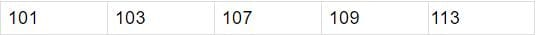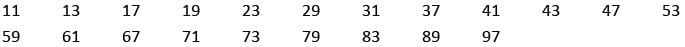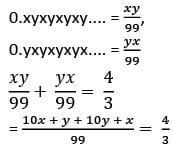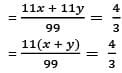# Arun Sharma Test: Number System- 3

## 15 Questions MCQ Test Quantitative Aptitude (Quant) | Arun Sharma Test: Number System- 3

Description
Attempt Arun Sharma Test: Number System- 3 | 15 questions in 20 minutes | Mock test for CAT preparation | Free important questions MCQ to study Quantitative Aptitude (Quant) for CAT Exam | Download free PDF with solutions
QUESTION: 1

### What is the sum in the Place value of 5 used in the following numbers: 567, 195, 573?

Solution:

► Place value of 5 in 567 = 5 x 100 = 500
► Place value of 5 in 195 = 5 x 1 = 5
► Place value of 5 in 573 = 5 x 100 = 500
► 500 + 5 + 500 = 1005

QUESTION: 2

### a, b, and c are 3 single-digit numbers such that 0.abcabcabc..... = 51/111. Find the value of a + b + c.

Solution:

► 0.abcabc.....is a non terminating recurring decimal number
► so as per the formula we discussed
⇒ 0.abcabc .... = abc/999 = 51/111,
⇒ abc = 51 x 9 = 459
► Since a, b and c are individual digits , a = 4; b = 5; and c = 9
⇒ a + b + c = 18

QUESTION: 3

### If abc is even, what would (a + b)(b + c)(c - a) would be?

Solution:

► For abc to be even there are three cases
► Case 1 : One of the three is Even
⇒ Let a be even , b and c be odd
⇒ (a + b)(b + c)(c - a) = (Even + Odd)(Odd + Odd)(Odd - Even)
⇒ Odd x Even x Odd = Even

► Case 2 : Two of three are Even
⇒ Let a and b be even and c be odd
⇒ (a + b)(b + c)(c - a) = (Even + Even)(Even + Odd)(Odd - Even)
⇒ Even x Odd x Odd = Even

► Case 3 : All three are Even
⇒ (a + b)(b + c)(c - a) = (Even + Even)(Even + Even)(Even - Even)
⇒ Even x Even x Even = Even

QUESTION: 4

If we subtract the square of two consecutive even numbers, by which natural number other than 1 would the difference be surely divisible by?

Solution:

► Since we know that the general expression for an even number is 2n, n being an Integer
► We are talking about two consecutive even numbers so,
let these numbers be 2n and 2(n+1)
► Difference of the square of two numbers would be [2(n+ 1)]2 − (2n)2
= 4(n2 + 1 + 2n) − 4n2
= 4n2 + 4 + 8n − 4n2
= 4 + 8n
= 4(2n + 1)

QUESTION: 5

What is the smallest positive Rational number?

Solution:

► Option A : 0 is not positive
► Rational numbers consist of fractions as well as Integers.
► Fraction can go to as small as possible.
► E.g. Even 0.5 is smaller than the given options of 1 and 2.

QUESTION: 6

What is the largest three digit prime number?

Solution:

► Option A : 991 when divided 6 gives a remainder of 1, It is a prime number
► Option B : 993 when divided 6 gives a remainder of 3, so not a prime number
► Option C : 997 when divided 6 gives a remainder of 1, it is a prime number
► Option D : 989 when divided 6 gives a remainder of 5, but it is divisible by 23

997 is greater than 991

QUESTION: 7

Which of the following is a prime number?

Solution:

► Option A : 5791 when divided 6 gives a remainder of 1, It is a prime number.
► Option B : 5793 when divided 6 gives a remainder of 3, so not a prime number.
► Option C : 5795 when divided 6 gives a remainder of 5, but is divisible by 5.
► Option D : 5792 when divided 6 gives a remainder of 2, so not a prime number.

QUESTION: 8

The sum of first five, 3 digit prime numbers is:

Solution:

► First 5, 3 digit prime numbers are listed below:► Their Sum is 533

QUESTION: 9

If a, (a+2), (a+4)  are all prime numbers, how many such values can 'a' take?

Solution:

► The given terms are: a, a + 2, a + 4
► For Less than 5, We know of such a set: 3, 5 and 7
► For Prime numbers Greater than 5 we have two cases
► Case 1 : The Prime number on division by 6 gives a remainder of 1
• Let us assume a is a prime number
• So a = 6p + 1
• a + 2 = 6p +3. (This will be divisible by 3. Thus not a prime)
• a + 4 = 6p + 7

► Case 2 : The Prime number on division by 6 gives a remainder of 5
• Let us assume a is a prime number
• So a = 6p + 5
• a + 2 = 6p + 7
• a + 4 = 6p + 9 (This will be divisible by 3. Thus not a prime.)

► Hence there is only one such set.

QUESTION: 10

What is the sum of all the negative two digit prime numbers?

Solution:

► Prime numbers is a property of natural numbers, There are no negative prime numbers.

QUESTION: 11

What is the negative of sum of all the two digit prime numbers?

Solution:

► All the two digit Prime numbers are listed below:►The sum is 1043
►The negative of this sum is - 1043

QUESTION: 12

For the equation (2a + 3b + 9c) to be even, we must have

Solution:

► Option A : b is Even
⇒ 2a + 3b + 9c = 2 x any number + 3 x Even + 9 x any number = Even + Even + Odd/Even
⇒ This does not give a sure shot result.

► Option B : c is Even
⇒ 2a + 3b + 9c = 2 x any number + 3 x any number + 9 x Even = Even + Odd/Even + Even
⇒ This does not give a sure shot result.

► Option D : Either of the two (b or c) is Even.
⇒ 2a + 3b + 9c = 2 x any number + 3 x Even + 9 x any number = Even + Even + Odd/Even
⇒ 2a + 3b + 9c = 2 x any number + 3 x any number + 9 x Even = Even + Odd/Even + Even
⇒ This does not give a sure shot result.

► Option C = Both b and c are Even
⇒ 2a + 3b + 9c = 2 x any number + 3 x Even + 9 x Even = Even + Even + Even = Even
⇒ So only option C gives an even response.

QUESTION: 13

What is the difference between the highest and smallest 3 digit prime number?

Solution:

► Highest 3 digit prime number = 997
► Smallest 3 digit prime number = 101
► Difference = 997 - 101 = 896

QUESTION: 14

0.xyxyxyxy.... + 0.yxyxyxyx = 4/3, if x and y are natural numbers what would be the value of x + y?

Solution:= x + y = 12

QUESTION: 15

What would the remainder when the square of the largest 4 digit prime number is divided by 6?

Solution:

► It is +1 with exceptions of prime numbers below 6 obviously.

► Any prime number above 6 is of the form:

6k + 1 or 6k - 1 ( A lot of people don't know this!))

► So therefore squares is of the form (6k ± 1)2. Expand this and clearly the only term which is not a multiple of 6 will be 1. Which again makes it of the form 6K + 1( now only + because we squared the whole term, Remember?)!.

► So therefore the remainder is of the form +1.

► So for every prime no. above 6, the remainder 1.

So, for 2, it is 4

For 3, it is 3.

For 5, it is 1.

► For every other prime no. Square, It’s 1.Use Code STAYHOME200 and get INR 200 additional OFF Use Coupon Code

### How to Prepare for CAT

Read our guide to prepare for CAT which is created by Toppers & the best Teachers